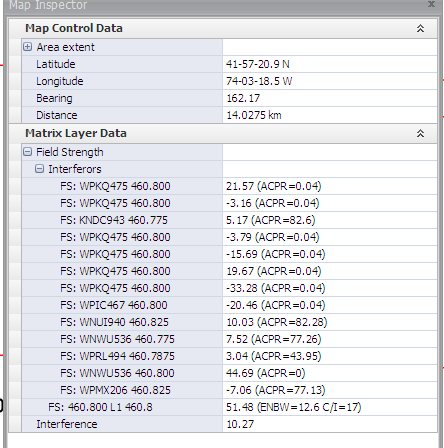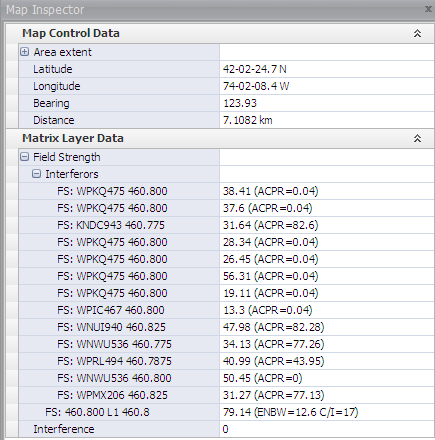# Map Inspector - Interference Studies

Submitted by Christo on Mon, 2013-07-22 10:43.
::

The map inspector gives information about the field strength (FS) of the proposed radio and all of the interfering radios at a given Lat/Lon point. This information is used to calculate the final value of interference, and can be used to determine who is causing the most of the interference at this point.

The interference calculations follow the following procedure:

At a given Lat/Lon point, we calculate the FS of the victim (the radio receiving interference), and the FS of all of the interfering radios. Based on the Frequency of the victim radio and the interferors, we calculate the adjacent channel protection ratios (ACPR). The calculations take into account the Equivalent Noise Bandwidth (ENBW) of the victim radio which depends on its modulation.

We extend the DeltaFrequency=Freq_victim – Freq_interferor with the ENBW, and the extended DeltaFrequency is then used to calculate the actual ACPR (see TSB88c for more details).

The ENBW is a measure of the frequency separation of the victim’s channels from the interferor’s channel. ACPR is a measure of how much protection the victim radio gets from a given interferor.

We adjust the FS of each interfering radio with the corresponding protection ratio, ACPR, and then we calculate the Equivalent interference FS of all of them at the point of interest. The Equivalent interference FS is of magnitude of the strongest interferor.
The interference value is calculated as:

Ix = FSEquivalent – Fsvictim + C/I, where C/I is the Carrier to Interference signal ratio, and in most cases in the range of 17dB.

The Ix values are in dB units.

All points where Ix>0 have interference and are represented with red color.

All points where Ix<=0 are not affected by interference, and we show them as having Ix=0 final value. They are shown on the map with green color.

----

Below are two examples of the Map inspector screen for a point with interference and a point with no interference.In this case, the FS of the victim is 51 dBu and the FS of the highest interferors is 44 with ACPR=0. The Interference value is ~10.At this point, the victim radio has FS of 79dBu, and the highest interferors has FS = 50dBu. There is no interference at this point.